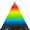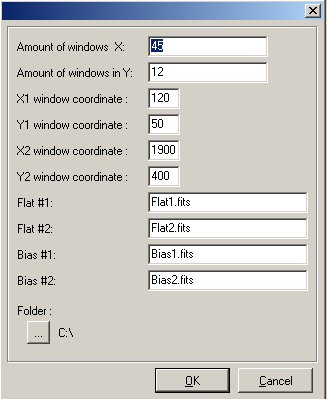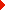> Analyse > Test CCD > Facteur de conversion

Lancement : Menu [Analyse] > [Test/Caractérisation de CCD] > [Facteur de conversion (multifenêtre)]

Cette fonction n'est pas accessible depuis la version light. Afin de pouvoir l'utiliser, il est nécessaire d'obtenir une clé "version complète" pour le logiciel.

But : Evaluer le facteur de conversion d'une caméra CCD à partir de plusieurs fichiers.

This method is very useful for computing the conversion factor during system development because it is fast and the accuracy is pretty good.

It just needs two biases and two flat field images. It performs conversion factor measurement using NxM sub windows to avoid any problems due to local defects. PRISM asks for the amount of windows that is needed across the X and Y axis. Note that subwindows less than 50x50 pixels can lead to false results, so for a 1x2K CCD for instance, set 10 windows for the X direction and 20 for the Y direction. To remove any prescan/overscan area set the X1,Y1,X2,Y2 windows so as to avoid them. then select the files.```Console output:
For all the windows (540) the results are the following :
window 1=4.49799e-/ADU X1=120 Y1=50 X2=158 Y2=78
window 2=4.11412e-/ADU X1=120 Y1=79 X2=158 Y2=107
window 3=4.31269e-/ADU X1=120 Y1=108 X2=158 Y2=136
window 4=4.14792e-/ADU X1=120 Y1=137 X2=158 Y2=165
window 5=4.0549e-/ADU X1=120 Y1=166 X2=158 Y2=194
..........
window 536=4.45689e-/ADU X1=1836 Y1=253 X2=1874 Y2=281
window 537=4.55087e-/ADU X1=1836 Y1=282 X2=1874 Y2=310
window 538=4.23658e-/ADU X1=1836 Y1=311 X2=1874 Y2=339
window 539=4.01395e-/ADU X1=1836 Y1=340 X2=1874 Y2=368
window 540=4.50764e-/ADU X1=1836 Y1=369 X2=1874 Y2=397
RMS noise =7.0774e- ± 0.092687
```

Method used :
Note that the algorithm supports flats fields that have different levels of illuminations, tests have been carried out with flats having means with factor of 50 between the two images and the feature passed it pretty well ! This method subtracts the biases from the flat field images, divides the two previous flat images and computes the RMS value (named N here) and the mean Signal for each window. Then computes the D=2*S/N^2 figure, from D, the bias noise is removed and yields to the conversion factor. The corrections due to the different flat field levels are performed by the software, but not mentioned in this explanation (to remain clear).

Focntion script associée :GetConvertFactor

Images de test :Images de testAucune